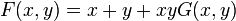# Commutative formal group law

## Definition

### One-dimensional formal group law

Let$R$ be a commutative unital ring. A one-dimensional formal group law on$R$ is a formal power series$F$ in two variables, denoted$x$ and$y$, such that:

Condition no. Name Description of condition
1 Associativity$\! F(x,F(y,z)) = F(F(x,y),z)$ as formal power series
2 Identity element$\! F(x,y) = x + y + xyG(x,y)$ for some power series$G$. Thus,$F(x,0) = x, F(0,y) = y$
3 Inverses There exists a power series$m(x)$ such that$m(0) = 0$ and$F(x,m(x)) = 0$

Note that conditions (1)-(3) alone define formal group law (which is not necessarily commutative). Also, condition (3) is redundant.

Two examples of commutative formal group laws, both of which work for any commutative unital ring, are the additive formal group law and the multiplicative formal group law.

### Higher-dimensional formal group law

Let$R$ be a commutative unital ring. A$n$-dimensional formal group law is a collection of$n$ formal power series$F_i$ involving$2n$ variables$(x_1,x_2,\dots,x_n,y_1,y_2,\dots,y_n)$ satisfying a bunch of conditions.

Before stating the conditions, we introduce some shorthand. Consider$x = (x_1,x_2,\dots,x_n)$ and$y = (y_1,y_2,\dots,y_n)$. Then,$F(x,y)$ is the$n$-tuple$(F_1(x_1,x_2,\dots,x_n,y_1,y_2,\dots,y_n),F_2(x_1,x_2,\dots,x_n,y_1,y_2,\dots,y_n),dots,F_n(x_1,x_2,\dots,x_n,y_1,y_2,\dots,y_n))$.

Condition no. Name Description of condition in shorthand Description of condition in longhand
1 Associativity$\! F(x,F(y,z)) = F(F(x,y),z)$ For each$i$ from$1$ to$n$,$F_i(x_1,x_2,\dots,x_n,F_1(y_1,y_2,\dots,y_n,z_1,z_2,\dots,z_n),F_2(y_1,y_2,\dots,y_n,z_1,z_2,\dots,z_n),\dots,F_n(y_1,y_2,\dots,y_n,z_1,z_2,\dots,z_n))$ equals$F_i(F_1(x_1,x_2,\dots,x_n,y_1,y_2,\dots,y_n),F_2(x_1,x_2,\dots,x_n,y_1,y_2,\dots,y_n),F_n(x_1,x_2,\dots,x_n,y_1,y_2,\dots,y_n),z_1,z_2,\dots,z_n)$.
2 Identity element$\! F(x,y) = x + y +$ terms of higher degree, so$\! F(x,0) = F(0,x) = x$ For each$i$,$\! F_i(x_1,x_2,\dots,x_n,y_1,y_2,\dots,y_n) = x_i + y_i +$ terms of higher degree (each further term is a product that involves at least one$x_j$ and one$y_k$.
3 Inverse There exists$m$, a collection of$n$ formal power series in one variable, such that$F(x,m(x)) = 0$ formally. There exist$m_i, 1 \le i \le n$, all formal power series in one variable, such that$\! F(x_1,x_2,\dots,x_n,m_1(x_1,x_2,\dots,x_n),m_2(x_1,x_2,\dots,x_n),\dots,m_n(x_1,x_2,\dots,x_n)) = 0$.

Note that conditions (1)-(3) define a (not necessarily commutative) formal group law. Condition (3) is redundant, i.e., it can be deduced from (1) and (2).